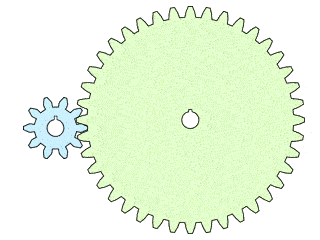# Gear Ratio Speed Calculator

Created by Rijk de Wet
Reviewed by Vishnuvardhan Shakthibala
Last updated: Apr 06, 2022

Welcome to the gear ratio speed calculator! Here, we'll show you:

• How to calculate the gear ratio and the speed at which your output gear rotates relative to your input gear; and
• How to calculate speed from the gear ratio.

## How do I use the gear ratio speed calculator?

The gear ratio speed calculator is easy to use and understand. Follow these simple steps:

1. Enter the number of teeth in each of your two gears. Make sure you know which is the driving gear and which one is driven!
2. Alternatively, to obtain the number of teeth for either gear for a suitable speed ratio, enter one gear's number of teeth and a suitable gear ratio instead.
3. Under the field for the gear ratio, we explain how the gear ratio influences the speed ratio between the input and output gears.
4. You can also enter an input speed to find the output speed corresponding to the two-gear system you've created above.

And that's it! You now know how to use the gear ratio speed calculator!

## How to calculate the gear ratio for gear speed?

When you have two connected gears, the gear ratio is the ratio between the input (driving) gear's number of teeth and the output (driven) gear's number of teeth. It allows the system designer to slow or speed up a rotational speed, which has other useful effects like influencing the torque. The gear ratio is also the factor by which the input speed is multiplied to deliver the output speed.

So, if you want to know how to calculate the gear ratio and the speed ratio mathematically, the equation would be:

$\footnotesize \text{Gear ratio} = \frac{ \text{\# teeth on input gear} }{ \text{\# teeth on output gear} }$

For example, a two-gear system featuring an input gear with $10$ teeth and an output gear with $20$ teeth would have a gear ratio of $10/20 = 0.5$, or $0.5:1$. The output speed would then be $0.5$ times faster (so $2$ times slower) than the input speed.

## The relationship between the gear ratio and the speed ratio

The speed ratio is all about angles. The input and output gears interlock, and so they each rotate one tooth at a time, together.

• If the output gear has more teeth than the input gear, the output gear has a smaller angle between teeth, resulting in a lower rotational speed on the output than the input. The gear speed ratio is lesser than 1.
• If the output gear has fewer teeth than the input gear, the angle between the output's teeth is larger, and the output gear rotates faster than the input. The gear speed ratio is greater than 1.

See the animation below: Having more teeth on the output (right) gear means a smaller angle to turn per tooth compared to the input (left) gear. This results in a gear speed ratio of <1, and a slower rotational speed on the output compared to the input.Haven't found quite what you're looking for with our gear ratio speed calculator? Why not try one of our other gear calculators:

## FAQ

### How do I calculate a vehicle's speed from its gear ratio?

To calculate the speed of the vehicle, follow these steps:

1. Determine the engine's speed in rotations per minute (rpm). Consult the car's tachometer for this value.
2. Multiply it with 3.6 × π × r, where r is the wheel radius in meters.
3. Divide it by 30 × g, where g is the gear ratio at the gearbox.
4. If the vehicle has a differential gear ratio, divide your result by it.

The result is the vehicle's speed in km/h.

### How do I calculate the speed ratio of gears?

The speed ratio is the inverse of the normal gear ratio. The gear ratio is the ratio of input teeth to output teeth (e.g., with 10 teeth on the input and 20 teeth on the output, the gear ratio is 10/20 or 0.5 : 1. The speed ratio is the ratio of output speed to input speed (e.g., with a gear ratio of 0.5, the output speed will be 0.5 that of the input speed).

### What is the speed ratio for a gear ratio of 0.5?

The output gear's speed will be 0.5 times as fast (so 2 times as slow) as the input's speed in a gear system with a total gear ratio of 0.5.

Rijk de Wet
Enter the number of teeth in your gears, and we'll work out the resulting gear speed ratio.
Input gear teeth
Output gear teeth
Gear ratio
:1
Input rotational speed
rpm
Output rotational speed
rpm
People also viewed…

### Bohr Model

The Bohr Model Calculator computes the frequency of emitted or absorbed electromagnetic wave at the transition of an electron between the orbits of an atom.

### Car heat

The hot car calculator shows you how fast the interior of a car heats up during a summers day.

### Plastic Footprint

Find out how much plastic you use throughout a year with this plastic footprint calculator. Rethink your habits, reduce your plastic waste, and make your life a little greener.

### Thermodynamic processes

Try this combined gas law calculator to find out the basic properties of the most common thermodynamic processes.